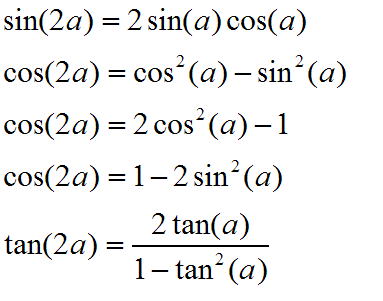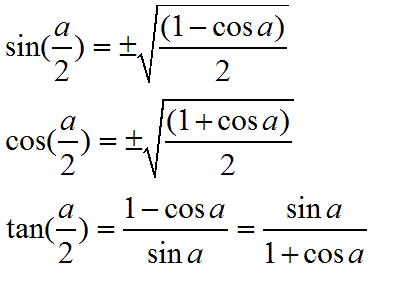# Solve Trig Problems With Double- or Half-Angles

The double and half angle formulas can be used to find the values of unknown trig functions. For example, you might not know the sine of 15 degrees, but by using the half angle formula for sine, you can figure it out based on the commonly known value of sin(30) = 1/2.

They are also useful for certain integration problems where a double or half angle formula may make things much simpler to solve.

## Double Angle Formulas:You'll notice that there are several listings for the double angle for cosine. That's because you can substitute for either of the squared terms using the basic trig identity $$\sin^2+\cos^2=1$$.

## Half Angle Formulas:These are a little trickier because of the plus or minus. It's not that you can use BOTH, but you have to figure out the sign on your own. For example, the sine of 30 degrees is positive, as is the sine of 15. However, if you were to use 200, you'd find that the sine of 200 degrees is negative, while the sine of 100 is positive. Just remember to look at a graph and figure out the sines and you'll be fine.